## 2.4 Polar Coordinates

There are two directions: the r direction is the direction of a vector from the origin to the point in question;
a unitĀ vector in this direction has representation:

ur = i cos+ j sinthedirection is normal to this:

u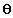= -i sin+ j cosThe vector r is represented in this coordinate system by r = rur , where r = (x2+y2)1/2;
since we have

r(t) = x(t) i + y(t) j

we obtain

x = rcos, y = rsinTaking derivatives we find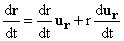verify by differentiating yourself that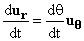which gives: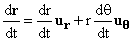We can compute the second derivative in polar coordinates by continued accurate use of the product and chain rules and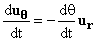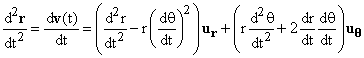The second and fourth terms here are sometimes referred to in physics as the centrifugal and Coriolis forces. Thus if an object is subject to no external force, so that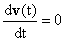you will find that it obeys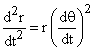,

and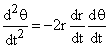The former causes the radial velocity to grow if there is angular motion: the latter slows down the angular motion if the object is moving away from the origin.